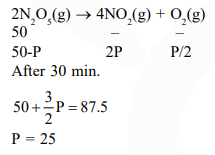Deepak Scored 45->99%ile with Bounce Back Crack Course. You can do it too!

# The reaction

Question:

The reaction

$2 \mathrm{~N}_{2} \mathrm{O}_{5}(\mathrm{~g}) \rightarrow 4 \mathrm{NO}_{2}(\mathrm{~g})+\mathrm{O}_{2}(\mathrm{~g})$

follows first order kinetics. The pressure of a vessel containing only $\mathrm{N}_{2} \mathrm{O}_{5}$ was found to increase from $50 \mathrm{~mm} \mathrm{Hg}$ to $87.5 \mathrm{~mm} \mathrm{Hg}$ in $30 \mathrm{~min}$. The pressure exerted by the gases after $60 \mathrm{~min}$.

1. $106.25 \mathrm{~nm} \mathrm{Hg}$

2. $116.25 \mathrm{~nm} \mathrm{Hg}$

3. $125 \mathrm{~mm} \mathrm{Hg}$

4. $150 \mathrm{~mm} \mathrm{Hg}$

Correct Option: 1

Solution: